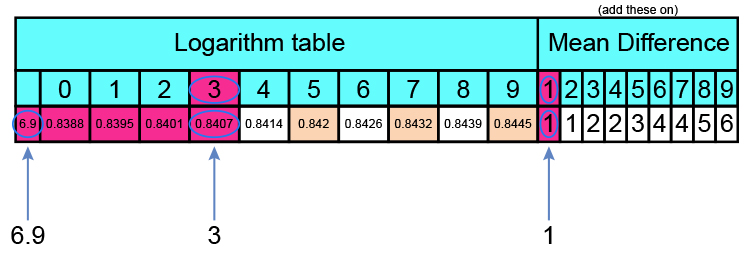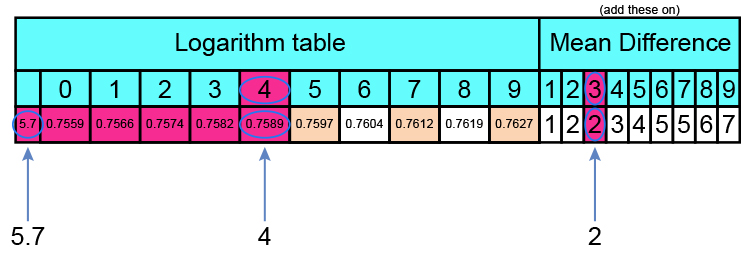# The log tables

So, how did we convert 69.31\times57.43 into 10^(1.8408)\times10^(1.7591) ?

We used log tables            (see reference log tables above)

Look up 69.31

Logs only work between 1 and 10

And so 69.31 is 10^1\times6.931

So we look up 6.931

Here is part of the log table:0.8407 + 1 (to the last digit) = 0.8408

Log table of 6.931 = 0.8408

So 69.31 = 10^1\times10^0.8408

69.31 = 10^1.8408 (adding indices)

Look up 57.43

Logs only work between 1 and 10

And so 57.43 is 10^1\times5.743

So we look up 5.743

Here is part of the log table:0.7589 + 2 (to the last digit) = 0.7591

Log table of 5.743 = 0.7591

So 57.43 = 10^1\times 10^0.7591

57.43 = 10^(1.7591) (adding indices)